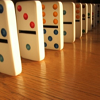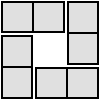#### You may also like### Consecutive Numbers

An investigation involving adding and subtracting sets of consecutive numbers. Lots to find out, lots to explore.### Domino Square

Use the 'double-3 down' dominoes to make a square so that each side has eight dots.### 4 Dom

Use these four dominoes to make a square that has the same number of dots on each side.

# Roll These Dice

##### Age 7 to 11 Challenge Level:

* I have found out that all the different possible answers are between $-4$ and $11$ including $11$ and $-4$. It is not possible to get any answers over $11$ and below $-4$.
I recorded all of this data in a table.
* I have found out that if all $3$ dice are the same the total will become the value of one of the die ( Eg. $1+1=2-1=1$ ).
* this would be the same conclusion as above if any $2$ of the die were the same. (E.g. $2+1=3-1=2$)
* I know there is a $50/50$ chance of the answer being odd or even because;
odd + odd - odd = odd
even + even - even = even
odd + even - odd = even
odd + odd - even = even
even + even - odd = odd
odd + even - even = odd
These are all the posible ways of adding the dice.
Thank you for reading my solution I hope all is correct.
Molly        ;-)
Indeed Molly it is very good and I am impressed that you did this and came to those conclusions. You could of course extend the exploration by wondering about using $4$ dice and deciding whether to subtract just $1$ of those or maybe $2$.

Ben, Harry, Will and Lucas from Tarporley Church of England School also worked on this activity and this was their report:

There are four of us so two of us wrote ALL of the combinations down [one from $6+6-1$ and one from $1+1-6$]. There were $216$ possible calculations. At the same time the other two of us worked out which is the most likely answer[which is $4$]. Once we did that we were done.

Sion from the same school added this extra piece of information;

There are $225$ ways and your answer is the numbers $3$ and $4$. By finding all the $225$ calculations you then make a tally chart to show the most popular number.  Finally you count up the number and then your answer should be $3$ and $4$.

We also had a number of good ideas from North Molton, namely, Michael, Jack, Beth, James and Sam.
Bram  from the British School of Bucharest  in  Romania , sent in what I think is the first from Romania, - well done and thanks - saying;

There is a higher probability to get $6$ than $2$ eg. there are fewer ways to get $2$ because there are $13$:

$1+2-1=2$ , $1+3-2=2$ , $1+4-3=2$ , $1+5-4=2$ , $1+6-5=2$ , $2+3-3=2$ , $2+4-4=2$, $2+5-5=2$ , $2+6-6=2$ , $3+3-4=2$ , $3+4-5=2$ , $3+5-6=2$ , $4+4-6=2$

and for $6$ there are:

$1+6-1=6$ , $2+5-1=6$ , $2+6-2=6$ , $3+4-1=6$ , $3+5-2=6$

Thanks you all, a great effort.